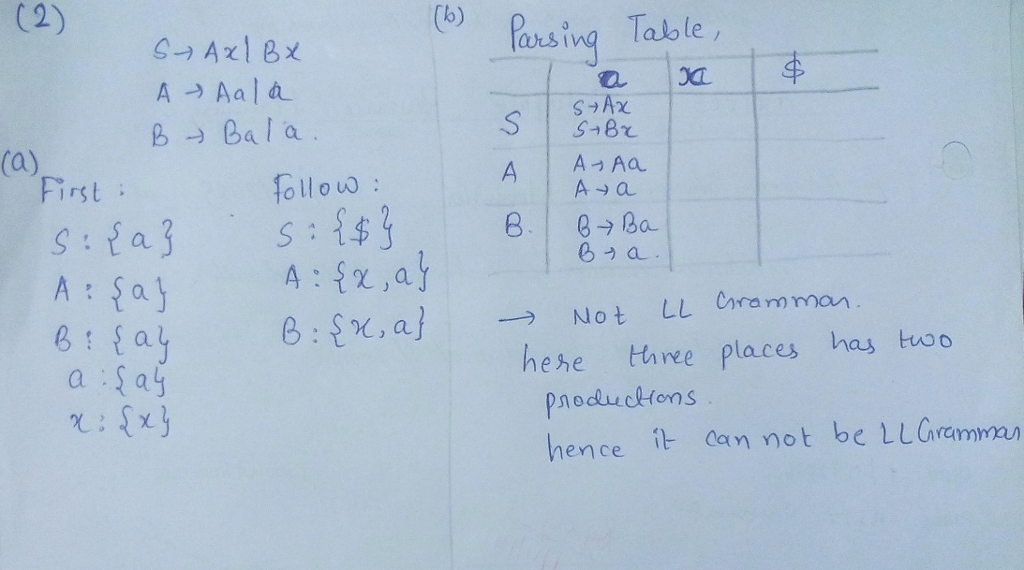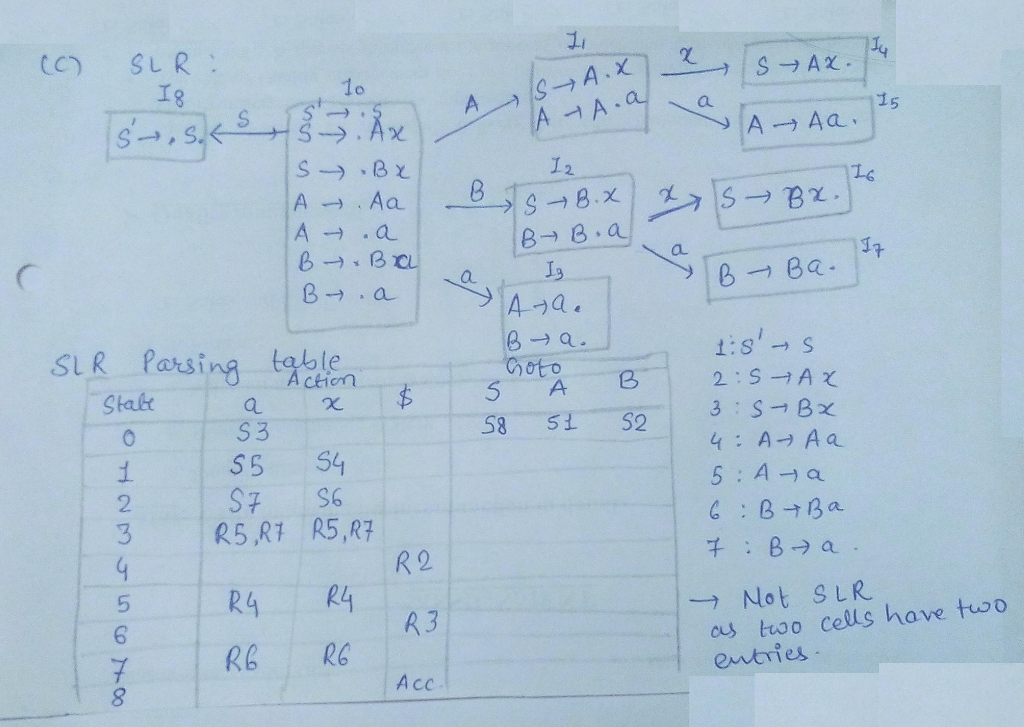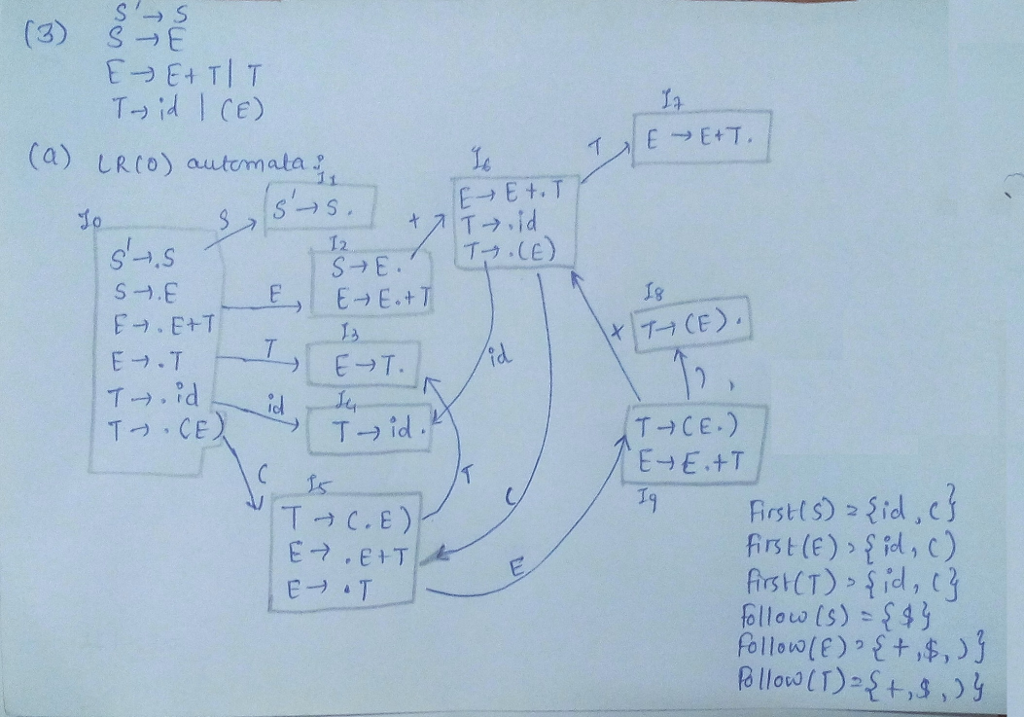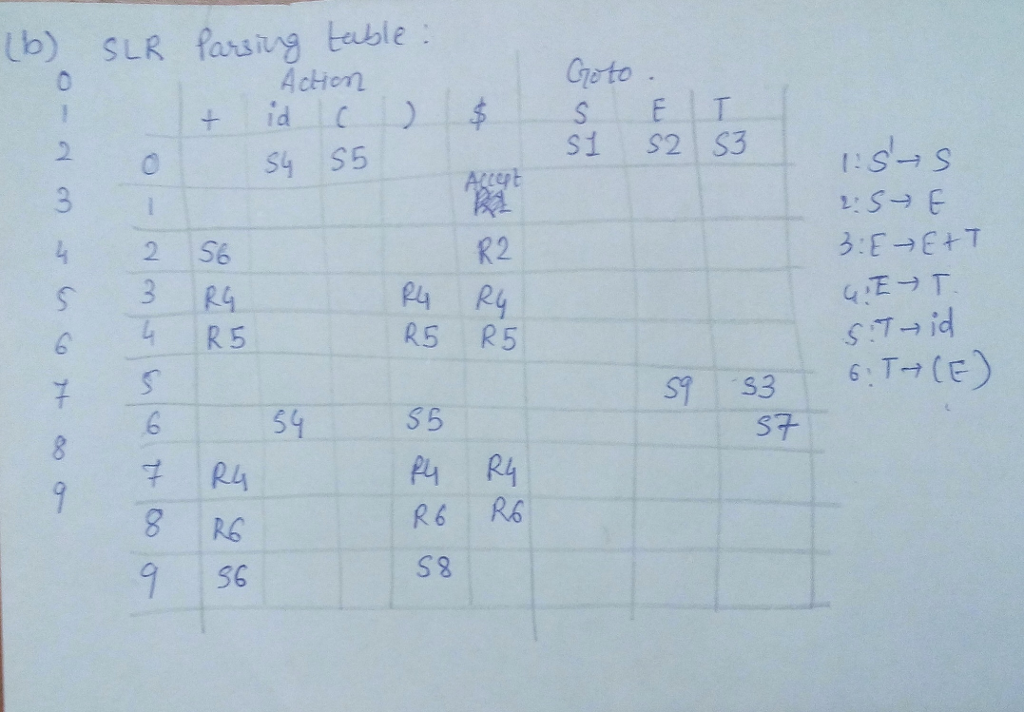# Answered! Given the following grammar G: S rightarrow Ax|By A rightarrow Aa|a B rightarrow Ba|a a) Compute the Follow set…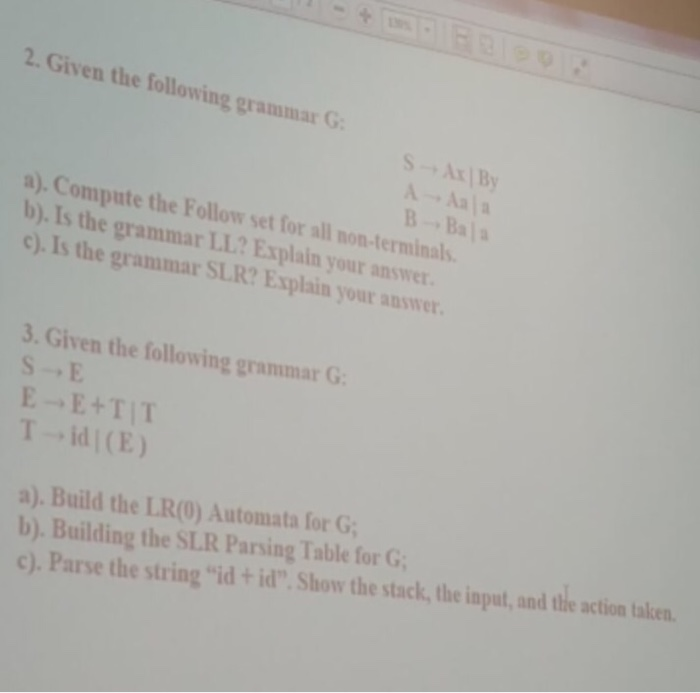Given the following grammar G: S rightarrow Ax|By A rightarrow Aa|a B rightarrow Ba|a a) Compute the Follow set for all non-terminals. b) Is the grammar LL? Explain your answer. c) Is the grammar SLR? Explain your answer. Given the following grammar G: S rightarrow E E rightarrow E + T|T T rightarrow id|(E) a) Build the LR(0) Automata for G: b) Building the SLR Parsing Table for G:c) Parse the string “id + id”. Show the stack the input, and the action taken.